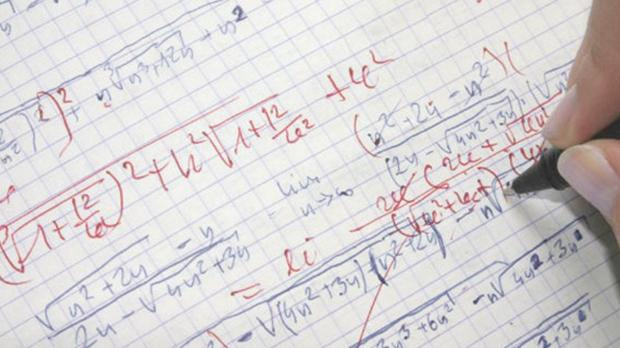Myth debunked: Maths is not a science
Advert

# Myth debunked: Maths is not a scienceMathematics is not a science.

The objective of science is to ask questions about the universe and attempt to answer them systematically through a scientific method. This entails formulating a hypothesis and searching for evidence, through observations and experiments which support that hypothesis. Conversely, mathematics relies on formal logical reasoning and deduction. Experimentation does of course inspire mathematicians: the infamous Goldbach conjecture, which states that every even number larger than two can be written as the sum of two primes, has been verified by computer for even numbers up to 4×1,018. But no mathematician will consider this to be a proof.

Science attempts to avoid assumptions about what it observes. Mathematics, on the other hand, is built entirely on assumptions. By combining assumptions, we can obtain new conclusions about the universe.

An example: suppose we have two assumptions: (i) 0 is an even number, and (ii) given any even number n, n+2 is also even. If we accept those, then we get that 0+2=2 is even. Consequently 2+2=4 is also even. The ‘mathematics’ here is not in the two assumptions but in the process of deducing additional facts. If we change the assumption (i) to (i’) ‘1 is an even number’, we get that 1+2=3 is even. It might seem strange that we conclude that 3 is an even number, but remember, it is not a fact of our universe, but of the universe defined by the assumptions (i’) and (ii).

Mathematics is often described as a science because scientists often make use of it and its language to model what they are studying. But as Georges Buffon said: “Mathematical truths are truths by definition. These definitions rest upon simple but abstract suppositions, and further truths are constructed of these definitions.”

Comments not loading? We recommend using Google Chrome or Mozilla Firefox with javascript turned on.
Comments powered by Disqus
Advert

## Popular Stories## Careers• ### Office Support Assistant• ### Junior Analyst (Passporting) within...• ### System AdministratorAdvert